##### Differential Equations For DummiesOptimization problems are one practical application of calculus problems. For example, optimization enables you to maximize your results (such as profit or area) with minimal input (such as cost or energy consumption).

The following practice question requires you to find the greatest volume for a soup can, using a given amount of tin.

## Practice question

1. What are the dimensions of the soup can of greatest volume that can be made with 50 square inches of tin? (The entire can, including the top and bottom, is made of tin.) And what's its volume?

1. The dimensions are 3 1/4 inches wide and 3 1/4 inches tall. The volume is about 27.14 cubic inches.

How do you find this? Start by drawing a diagram.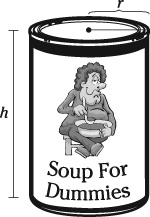Now write a formula for the thing you want to maximize — in this case, the volume: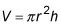Use the given information to relate r and h.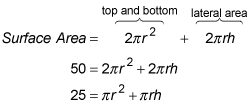Now solve for h and substitute to create a function of one variable.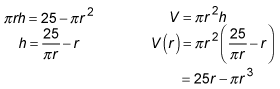Figure the domain.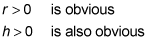And because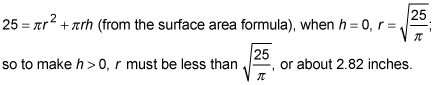Now find the critical numbers of V (r).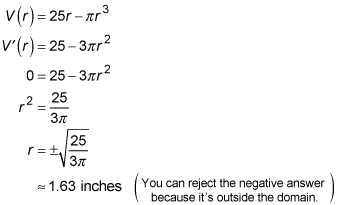Evaluate the volume at the critical number.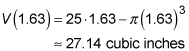That's about 15 ounces. The can will be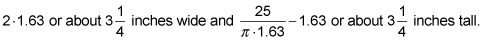Isn't that nice? The largest can has the same width and height and would thus fit perfectly into a cube. Geometric optimization problems frequently have results where the dimensions have some nice, simple mathematical relationship to each other.